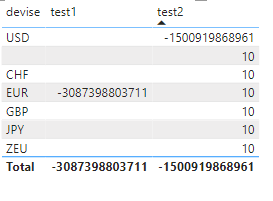cancel
Showing results for
Did you mean:Frequent Visitor

## CALCULATE with KEEPFILTERS not working when adding constant

Hello 🙂

I am facing an issue on my visual.

I have created two measures :

test1 = CALCULATE(Mesures[Encours_1],KEEPFILTERS('Dimension Divers'[devise]="EUR"))
test2 = CALCULATE(Mesures[Encours_1]+10,KEEPFILTERS('Dimension Divers'[devise]="USD"))

But as you can see below.. As soon as we add a constant number to the CALCULATE the KEEPFILTERS function is not working.
It puts the value everywhere...I have do some further testing and it seems that as soon as we do an addition in the CALCULATE then it won't work (but it works for a multiplication !). I have also tried to put the addition after the CALCULATE but same issue..

Do you have any idea ?

Romain

1 ACCEPTED SOLUTIONSuper User

Try

``````test2 =
CALCULATE (
Mesures[Encours_1]
+ IF ( SELECTEDVALUE ( 'Dimension Divers'[devise] ) = "USD", 10 ),
KEEPFILTERS ( 'Dimension Divers'[devise] = "USD" )
)
``````Super User

Try

``````test2 =
CALCULATE (
Mesures[Encours_1]
+ IF ( SELECTEDVALUE ( 'Dimension Divers'[devise] ) = "USD", 10 ),
KEEPFILTERS ( 'Dimension Divers'[devise] = "USD" )
)
``````